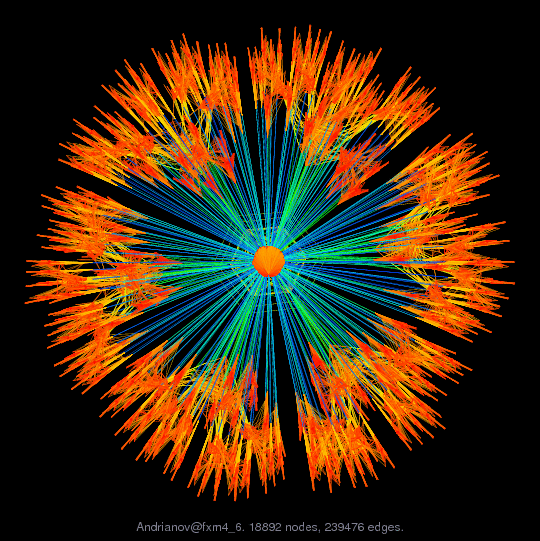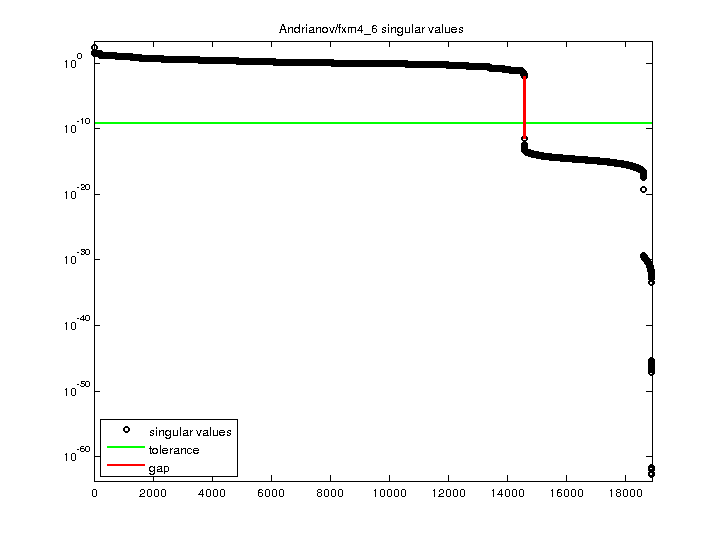Matrix: Andrianov/fxm4_6

Description: fxm4_6 matrix from Alexander Andrianov, SAS Institute Inc.(undirected graph drawing)• Matrix group: Andrianov
• download as a MATLAB mat-file, file size: 369 KB. Use UFget(1381) or UFget('Andrianov/fxm4_6') in MATLAB.

 Matrix properties number of rows 18,892 number of columns 18,892 nonzeros 497,844 structural full rank? yes structural rank 18,892 # of blocks from dmperm 1 # strongly connected comp. 1 explicit zero entries 0 nonzero pattern symmetry symmetric numeric value symmetry symmetric type binary structure symmetric Cholesky candidate? yes positive definite? no

 author A. Andrianov editor T. Davis date 2006 kind optimization problem 2D/3D problem? no

 Ordering statistics: result nnz(chol(P*(A+A'+s*I)*P')) with AMD 364,975 Cholesky flop count 1.3e+07 nnz(L+U), no partial pivoting, with AMD 711,058 nnz(V) for QR, upper bound nnz(L) for LU, with COLAMD 619,679 nnz(R) for QR, upper bound nnz(U) for LU, with COLAMD 1,441,462

 SVD-based statistics: norm(A) 228.084 min(svd(A)) 1.90802e-63 cond(A) 1.19539e+65 rank(A) 14,569 sprank(A)-rank(A) 4,323 null space dimension 4,323 full numerical rank? no singular value gap 2.86106e+09

 singular values (MAT file): click here SVD method used: s = svd (full (R)) ; where [~,R,E] = spqr (A) with droptol of zero status: ok